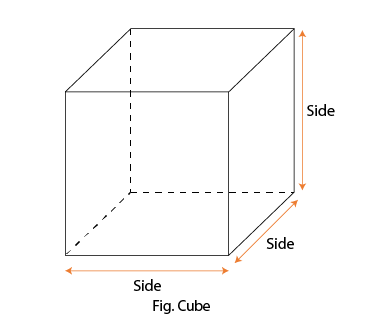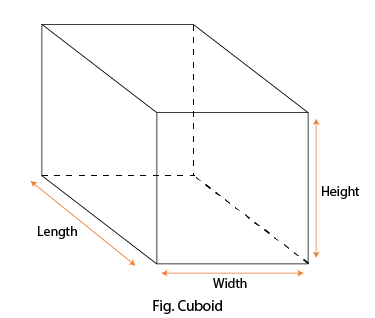Algebraic Identity (a + b)³

## Objective

To verify the algebraic identity: (a + b)³ = a³ + b³ + 3a²b + 3ab².

### Pre-requisite Knowledge

• Concept of the cube
• Concept of the cuboid
• Multiplication of numbers
• Prior knowledge of the volume of cubes or cuboids

### Concept

• An Algebraic Identity is equality, which is true for all the values of the variables in equality. They are also used for the factorization of polynomials. The algebraic equations that are valid for all values of the variables in them are called algebraic identities.
• In this way, algebraic identities are used in the computation of algebraic expressions and in solving different polynomials.

### Cube

A cube is a region of space formed by six identical square faces joined along their edges. Three edges join at each corner to form a vertex.

### Volume of the cube

• The volume of the cube is the space contained in it. Suppose an object is cubical and we need to immerse any material in it, say water, then the measure of water in litres to be kept in the object is calculated by its volume.
• The formula for the volume is given by: Volume of cube = (side)³ (cubic units). Refer to Fig. Cube.### Cuboid

A cuboid is a 3D shape with rectangular sides. Cuboids have six surfaces and twelve edges. Objects that are cuboid include books, matchboxes, and shoe boxes. If a cuboid has faces that are all square, it is a cube. All the angles of a cuboid are right angles.

### Volume of the cuboid

• The volume of a cuboid is the total space occupied by the cuboid in three-dimensional space. A cuboid is a three-dimensional structure with six rectangular faces. These six faces of the cuboid exist as a pair of three parallel faces.
• Therefore, volume is a measure based on the dimensions of these faces, i.e., length, width, and height.
• Volume of a cuboid = length × width × height (cubic units). Refer to Fig. Cuboid.